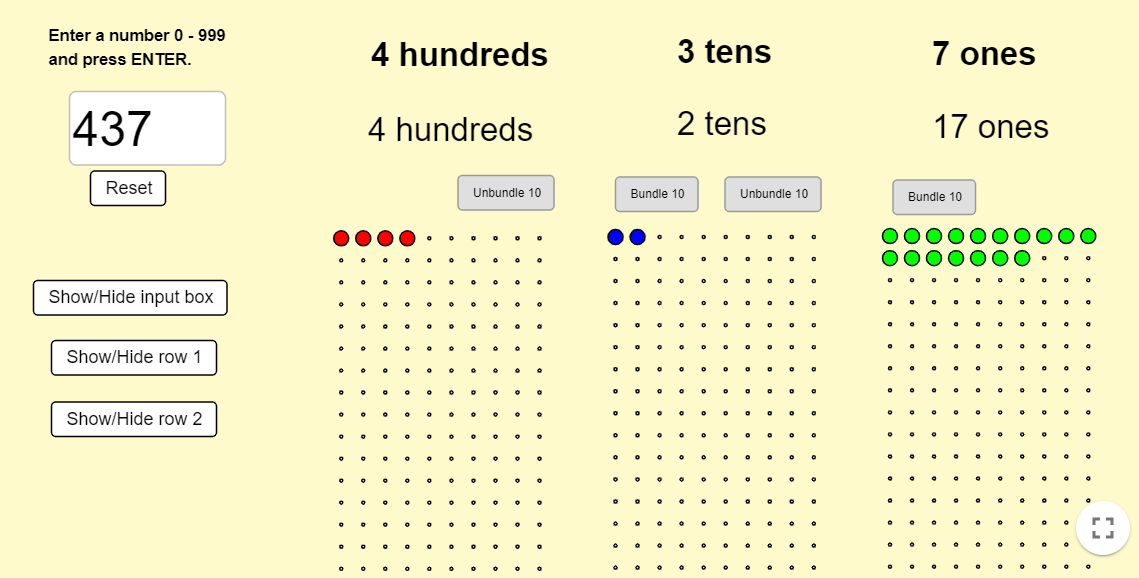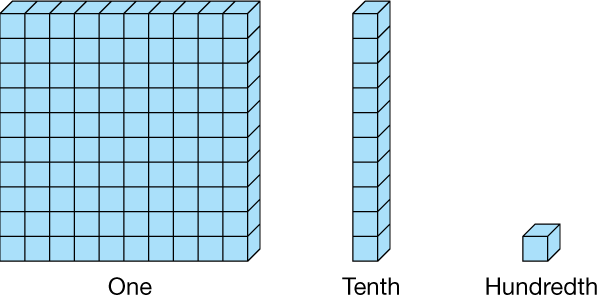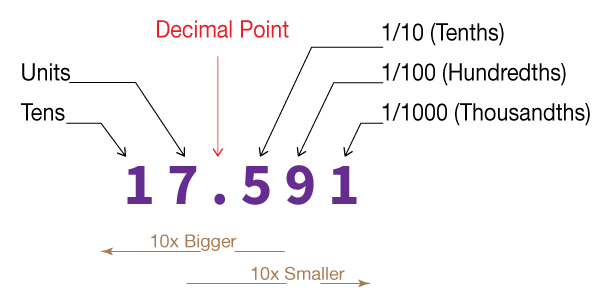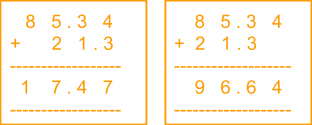# Addition and Subtraction of Decimals

Go back to  'Decimals'

## Introduction to Addition and Subtraction of Decimals

Today we will be learning how to add or subtract decimals. There’s nothing to be scared of, this is EXACTLY like the addition and subtraction of whole numbers that you can now do in your sleep!

## The Big Idea: The Place Value

The one key idea that needs to be highlighted before starting off with operations on decimals is that of Place Value.

When you look at the number 145 you know there are three digits in each, and these are the place value of the three digits:

\begin{align} &{\rm{1}}\;{\rm{ = }}\;{\text{hundreds place}}\\ &{\rm{4}}\;{\rm{ = }}\;{\text{tens place}}\\ &{\rm{5}}\;{\rm{ = }}\;{\text{units place}}\\ &{\text{That is why we can write 145 as}}\\ &{\rm{145}}\;{\rm{ = }}\;\left( {{\rm{1}} \times {\rm{100}}} \right){\rm{ + }}\left( {{\rm{4}} \times {\rm{10}}} \right){\rm{ + }}\left( {{\rm{5}} \times {\rm{1}}} \right)\;\\ &{\rm{ = }}\;{\rm{100}}\;{\rm{ + }}\;{\rm{40}}\;{\rm{ + }}\;{\rm{5}}\;{\rm{ = }}\;{\rm{145}} \end{align}

Here’s a neat way to try and picture the place values of digits:### Place Values for decimals

While considering decimals or numbers to the right of the point, the convention remains the same, however, flipped. With every place you move to the right, the place value gets divided by 10 instead of multiplied. So:Basically, if we were to write the number 0.145, these would be the place values of each digit:

\begin{align} &{\rm{0}}{\rm{.145}}\;{\rm{-}}\\ &{\rm{1}}\;{\rm{ = }}\;{\rm{tenth}}\\ &{\rm{4}}\;{\rm{ = }}\;{\rm{hundredth}}\\ &{\rm{5}}\;{\rm{ = }}\;{\rm{thousandth}} \end{align}

So, we can write 0.145 as

\begin{align} &\left( {1 \times \frac{1}{{10}}} \right) + \left( {4 \times \frac{1}{{100}}} \right) + \left( {5 \times \frac{1}{{1000}}} \right)\\ & = \left( {1 \times 0.1} \right) + \left( {4 \times 0.01} \right) + \left( {5 \times 0.001} \right)\\ & = 0.1 + 0.04 + 0.005\\ & = 0.145 \end{align}

If you’re still having doubts, here’s another useful image that will help you see the change of place values on the left and the right of the decimal point:### The Equivalence of decimals

The last thing you need to understand before you go on to the addition and subtraction of decimals is that adding of zeroes to the left of a whole number and to the right of decimals does not change the number at all. So, you can write 56 as 056 without any change in value, and you can also write 0.56 as 0.560 without any change in value.

## How is it important?

### Addition and subtraction of decimals

The simplest rule to remember about using operators on decimals is that the decimal point of all the numbers being added or subtracted needs to be aligned in a straight line perfectly. This means that each of the place values on either side of the decimal point would be exactly below each other. In other words, the hundredth place of both numbers should be right above/below each other and so should the thousandth place. Once that is done, the addition or subtraction would be done exactly like the adding or subtracting of two whole numbers.

Let us look at how we add two number 345.298 and 46.376. The wrong way to write these two numbers would be this:

\begin{align} & ~3\ 4\ 5.2\ 9\ 8 \\ & \underline{+\ 4\ 6.3\ 7\ 6} \\ & \underline{\ \ \ \ \ \ \ \ \ \ \ \ \ \ \ \ \ \ \,} \\ \end{align}

As we said, the decimal points of both numbers need to be aligned, so this is how you would write them:

\begin{align} & ~3\ 4\ 5.2\ 9\ 8 \\ & \underline{+\ 4\ 6.3\ 7\ 6} \\ & \underline{\,~3\ 9\ 1.6\ 7\ 4} \\ \end{align}

If we had to subtract them that too would play out perfectly only if the decimal places were perfectly aligned.

\begin{align} & ~3\ 4\ 5.2\ 9\ 8 \\ & \underline{-\ 4\ 6.3\ 7\ 6} \\ & \underline{\ 2\ 9\ 8.9\ 2\ 2} \\ \end{align}

One last tip is that if one of the two numbers is a whole number you need to place the decimal point after it and then follow with as many zeroes are required. Similarly, a decimal number less than one can have as many zeroes added to the left of the decimal point as required.

## Common mistakes or misconceptions

• Children place numbers incorrectly when adding or subtracting. This happens mostly because they have memorised the fact that when adding the rightmost digits of the two numbers need to be one below the other. This works for whole numbers but does not work for decimals.• When adding decimals which are measurements, children may incorrectly write down a decimal number. This means any subsequent operation will also be incorrect.
E.g. children may write 1 km and 5 meters as 1.5 km instead of 1.005 km

## Tips and Tricks

• Tip: When adding or subtracting decimal numbers, align the two numbers such that the decimal points are one below the other. This will ensure the place values match.

When comparing two decimal numbers, insert trailing zeros (zeros at the end of a number after the decimal point) if the number of digits after zero are fewer in a particular number. E.g. When adding 1.56 and 4.329, rewrite 1.56 as 1.560. This ensures that both decimals have an equal number of digits after the decimal point. This will make adding or subtracting easier.

More Important Topics
Numbers
Algebra
Geometry
Measurement
Money
Data
Trigonometry
Calculus
More Important Topics
Numbers
Algebra
Geometry
Measurement
Money
Data
Trigonometry
Calculus
Learn from the best math teachers and top your exams

• Live one on one classroom and doubt clearing
• Practice worksheets in and after class for conceptual clarity
• Personalized curriculum to keep up with school

0